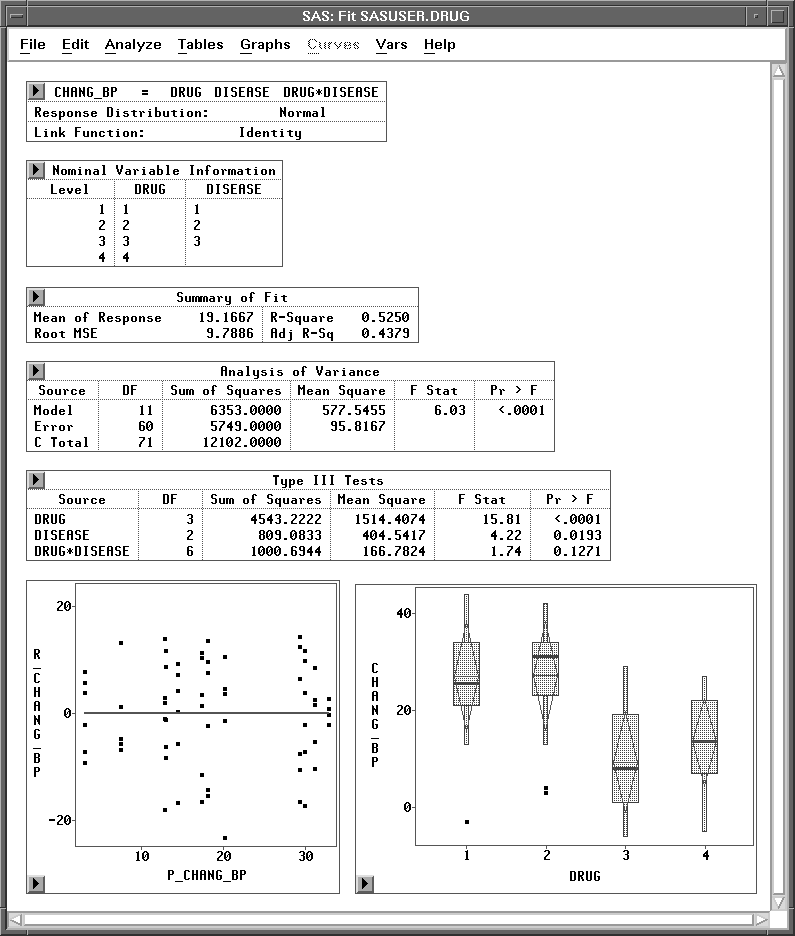Previous Page Next Page
 Analysis of Variance

# Analysis of Variance

In this chapter, you consider analyses that use least-squares methods to fit the general linear model. Such analyses include regression, analysis of variance, and analysis of covariance. You can choose Analyze:Fit (Y X) to carry out an analysis of variance. You can use box plots to examine individual group means.Figure 15.1: Fit Window with Analysis of Variance Results

#### References

 Previous Page Next Page Top of Page